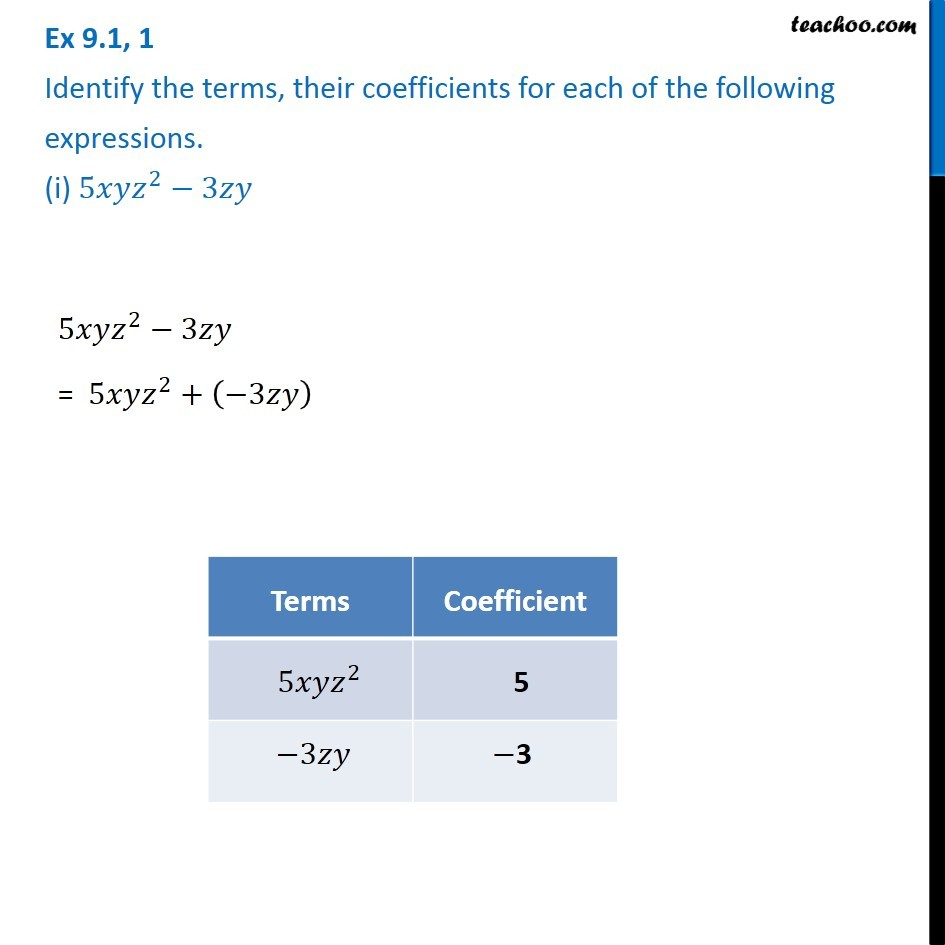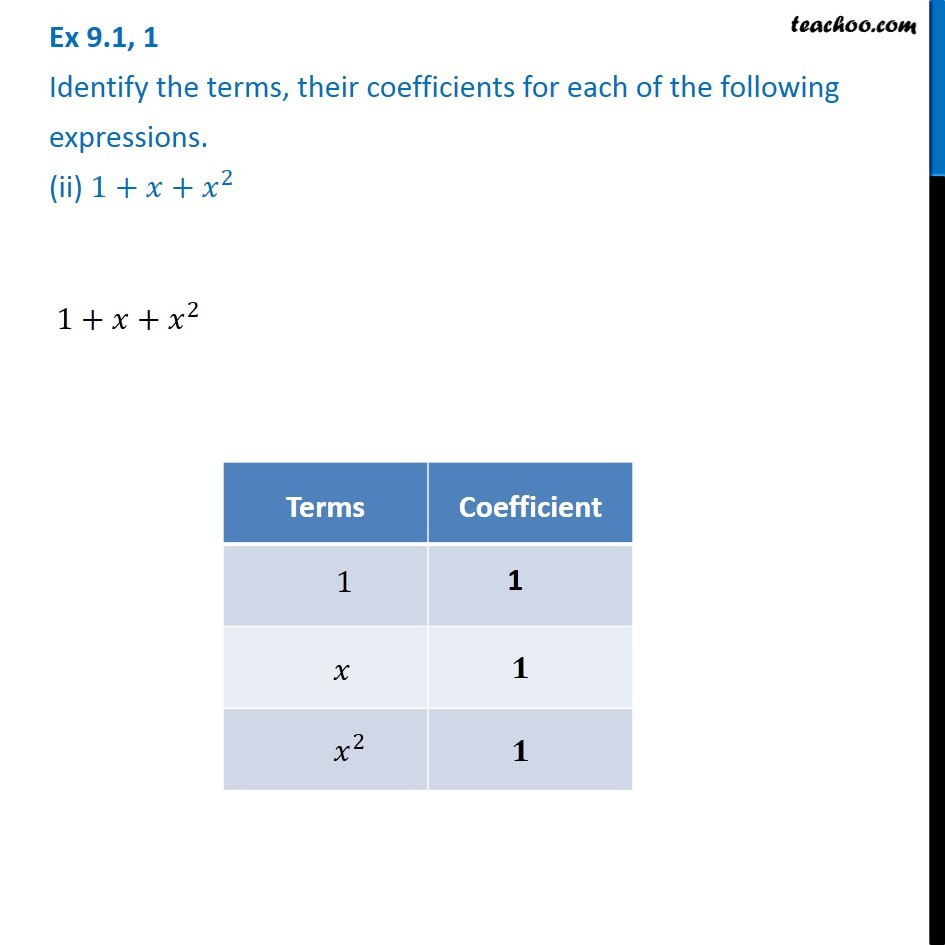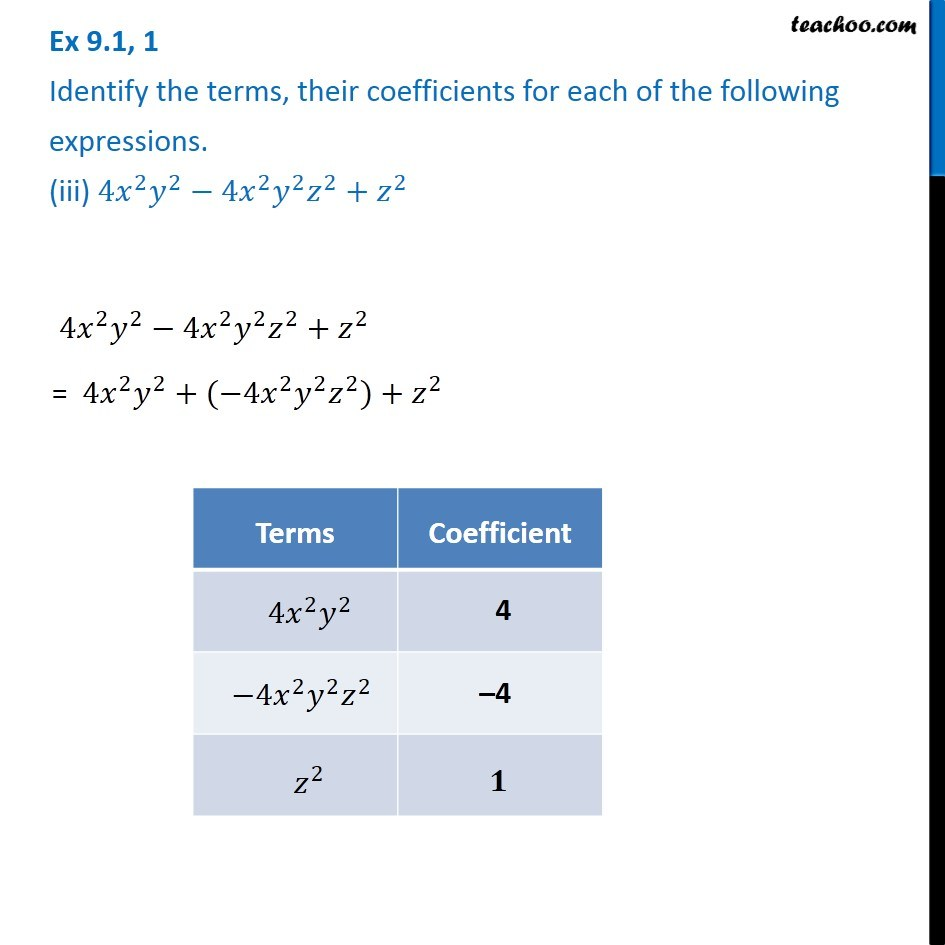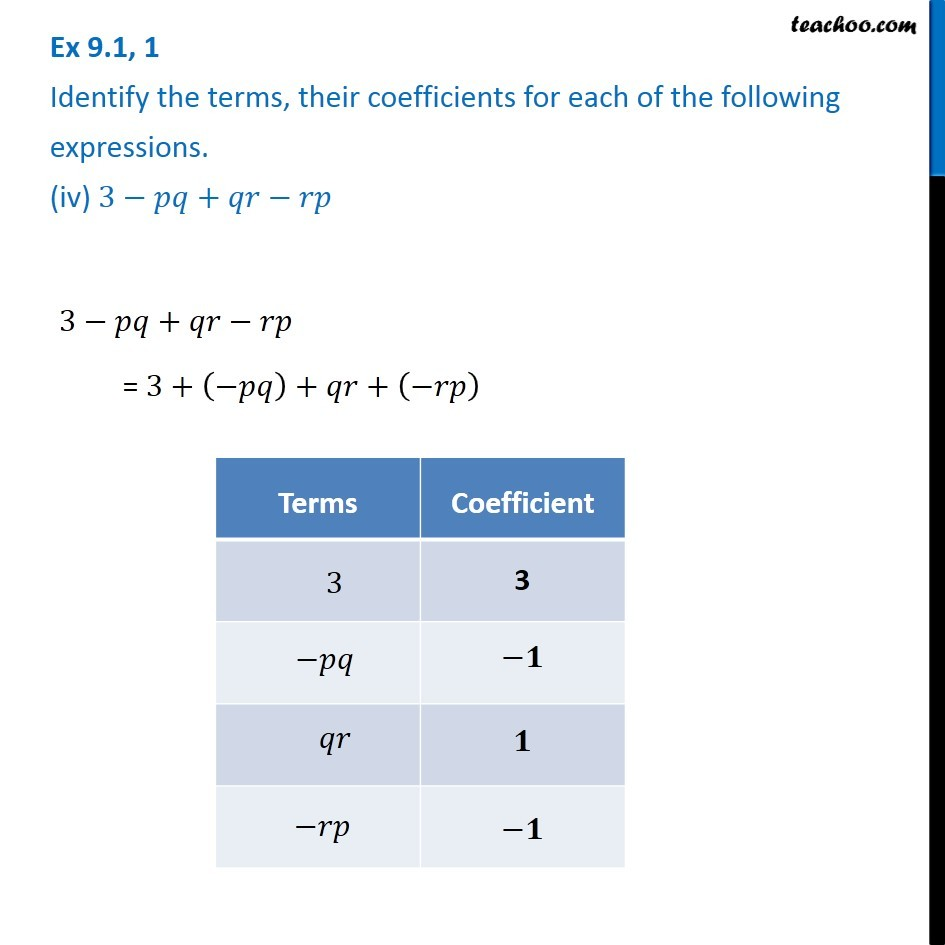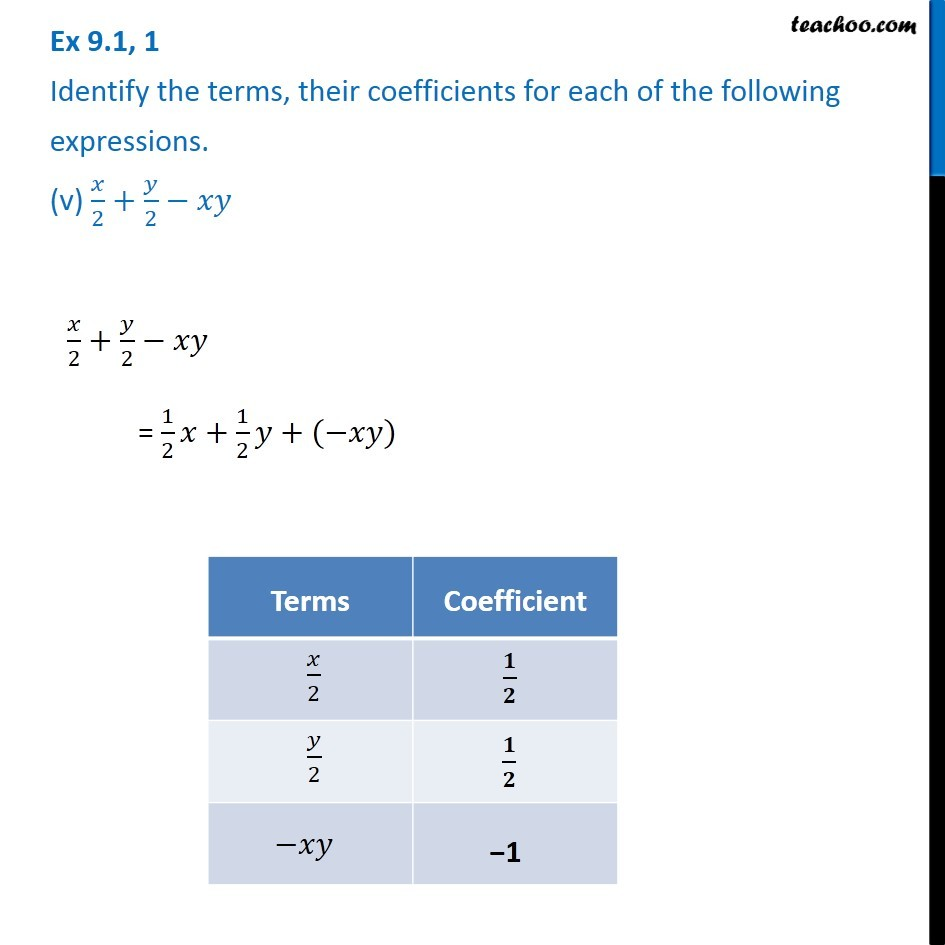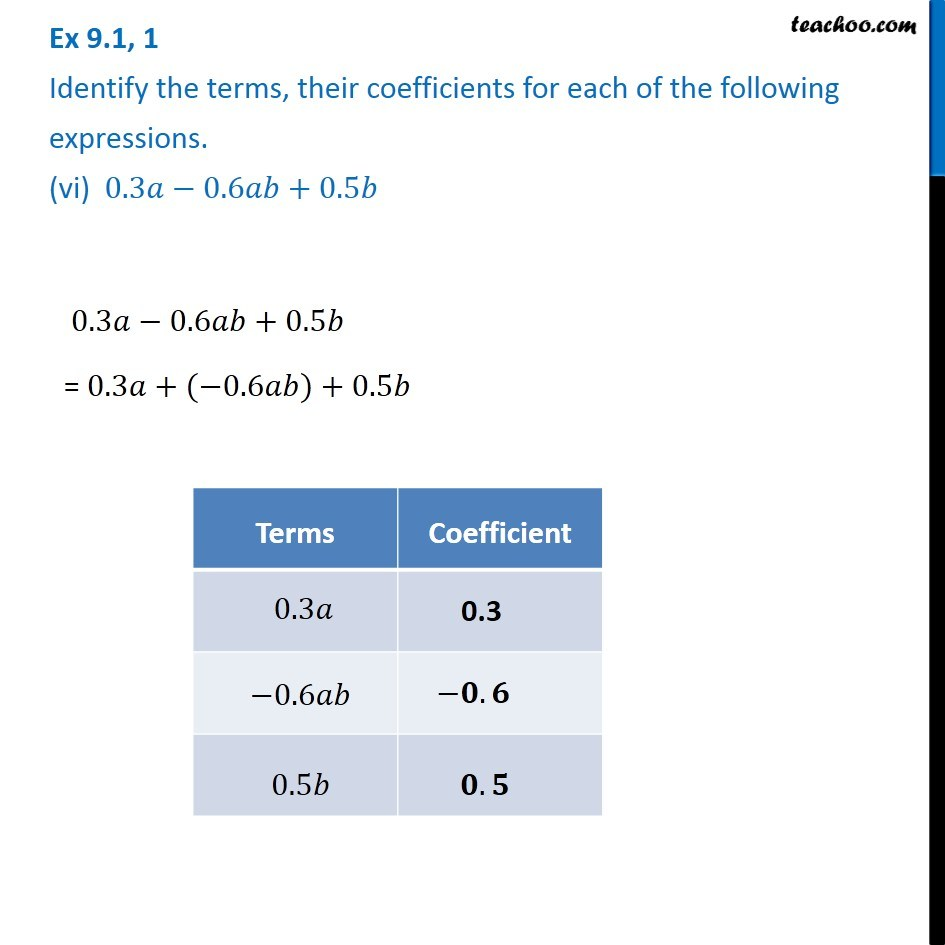Subscribe to our Youtube Channel - https://you.tube/teachoo

1. Chapter 9 Class 8 Algebraic Expressions and Identities
2. Serial order wise
3. Ex 9.1

Transcript

Ex 9.1, 1 Identify the terms, their coefficients for each of the following expressions. (i) 5𝑥𝑦𝑧^2−3𝑧𝑦 5𝑥𝑦𝑧^2−3𝑧𝑦 = 5𝑥𝑦𝑧^2+(−3𝑧𝑦) Ex 9.1, 1 Identify the terms, their coefficients for each of the following expressions. (ii) 1+𝑥+𝑥^2 1+𝑥+𝑥^2 Ex 9.1, 1 Identify the terms, their coefficients for each of the following expressions. (iii) 4𝑥^2 𝑦^2−4𝑥^2 𝑦^2 𝑧^2+𝑧^2 3−𝑝𝑞+𝑞𝑟−𝑟𝑝 = 3+(−𝑝𝑞)+𝑞𝑟+(−𝑟𝑝) Ex 9.1, 1 Identify the terms, their coefficients for each of the following expressions. (v) 𝑥/2+𝑦/2−𝑥𝑦 𝑥/2+𝑦/2−𝑥𝑦 = 1/2 𝑥+1/2 𝑦+(−𝑥𝑦) Ex 9.1, 1 Identify the terms, their coefficients for each of the following expressions. (vi) 0.3𝑎−0.6𝑎𝑏+0.5𝑏 0.3𝑎−0.6𝑎𝑏+0.5𝑏 = 0.3𝑎+(−0.6𝑎𝑏)+0.5𝑏

Ex 9.1